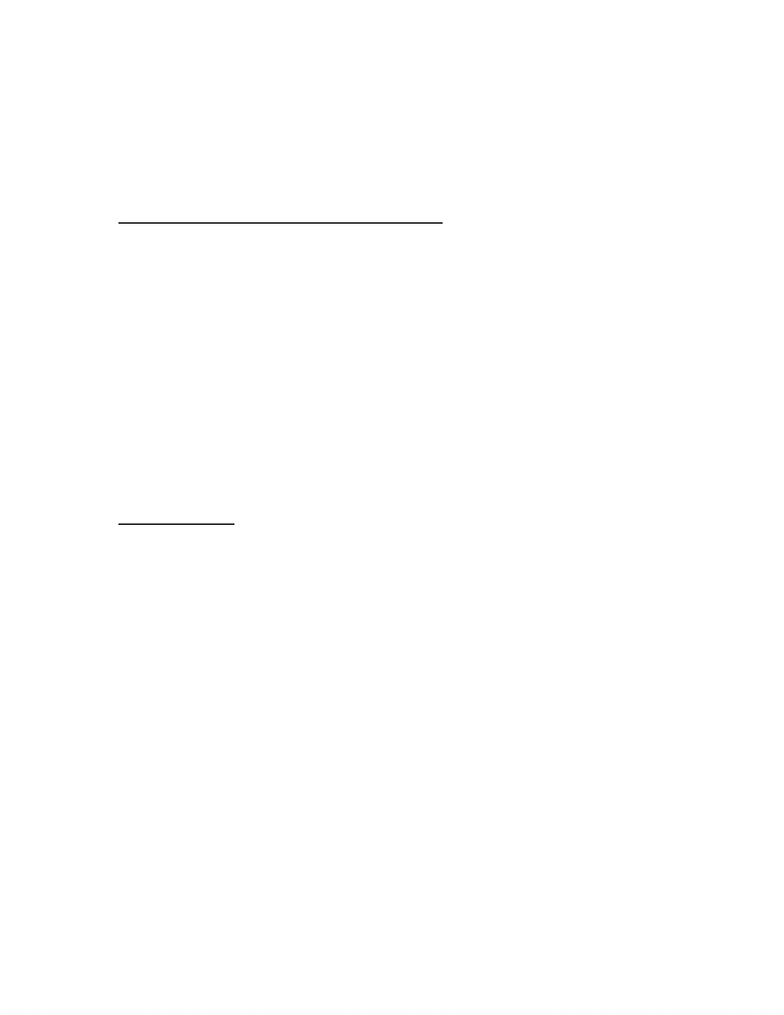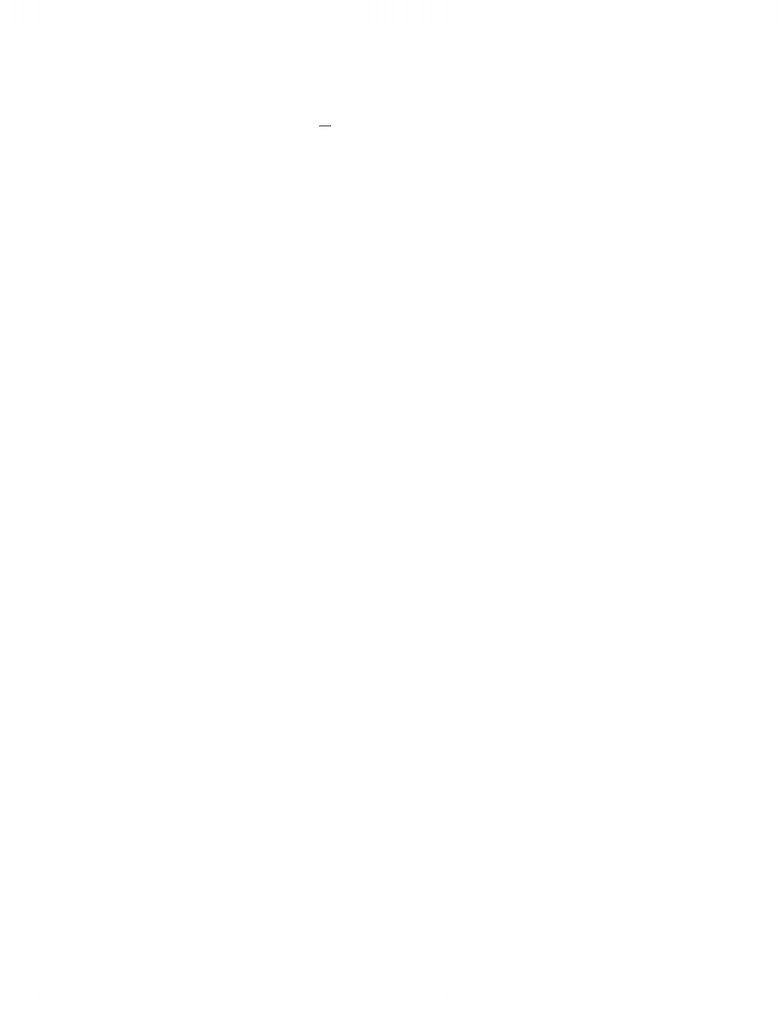# ACC410 Solution Chapter 2

106 views5 pagesChapter 2
The Cost Function
MULTIPLE-CHOICE QUESTIONS
2.10
Ans: A
2.11
Ans: B
2.12
Ans: B
2.13
Ans: C
2.14
Ans: D
EXERCISES
2.16
A. Variable cost per car wash = 5.25
Total fixed costs = 115,000
Cost Equation TC = 115,000 + 5.25*number of car washes
B. TC = 203,200
C. Variable Cost / unit = 5.41
Total Fixed Cost = \$ 118,310
Cost Equation TC = 215,690
2.19
A. TC = \$10,000 + \$8.00*Q
B. TC = \$25,000 + \$8.00*Q, for Q 2,000
TC = \$35,000 + \$8.00*Q, for Q > 2,000
C. Estimated cost per unit = \$43
D. TC = \$9,500
Unlock document

This preview shows pages 1-2 of the document.
Unlock all 5 pages and 3 million more documents.2-2 Cost Management
2.20
A. TC = \$50,000 + \$10.00*Q, for Q < 1,000
TC = \$51,000 + \$9.00*Q, for Q > 1,000
2.21
A. cumulative average time = 4.8 hours (average time for each of the next two returns)
2.22
A. TC = \$20,000 + 25%*Total sales
2.23
A. D or I, F
B. D, V
C. I, F
D. D or I, V
E. I, V or F
F. I, F or M
G. I, F
H. I, F
I. I, F or M
J. D or I, V
2.24
A. Total Fixed = 3,300
Total Variable = 4,500
B. TC = \$3,300 + 50%*Total revenue
C. lost contribution margin from the products sold with the flavour that has been replaced.
D.irrelevant
2.25
A. B; F, V, or M
B. PS; V
C. B; F or M
D. FB; F
E. B; F
F. PS; F
G. B; F or M
2.26
A. Cost of plain burger = \$0.3489
B. Cost of burger with everything except cheese = \$0.5196
Suggested selling price = \$1.56
Unlock document

This preview shows pages 1-2 of the document.
Unlock all 5 pages and 3 million more documents.